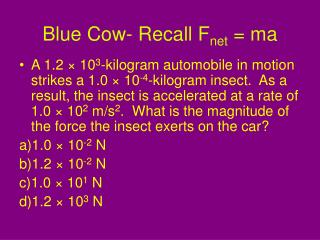DownloadDownload PresentationBlue Cow- Recall F net = ma

# Blue Cow- Recall F net = ma

Download Presentation## Blue Cow- Recall F net = ma

- - - - - - - - - - - - - - - - - - - - - - - - - - - E N D - - - - - - - - - - - - - - - - - - - - - - - - - - -
##### Presentation Transcript

1. Blue Cow- Recall Fnet = ma A 1.2 × 103-kilogram automobile in motion strikes a 1.0 × 10-4-kilogram insect.  As a result, the insect is accelerated at a rate of 1.0 × 102 m/s2.  What is the magnitude of the force the insect exerts on the car? 1.0 × 10-2 N 1.2 × 10-2 N 1.0 × 101 N 1.2 × 103 N

2. Objective • Explore what causes motion ! Apply Newton’s laws of motion to the world around us.

3. Homework • Castle Learning – Newton 1 • Possible Test Next Friday.

4. Force • Force is a vector quantity, therefore it has both magnitude and direction. • The SI unit for force is the Newton. • The Newton is a derived unit where: 1N = 1kg•m/s2 • 1 Newton is the amount of force required to accelerate a 1 kg mass 1 m/s2.

5. FN System Environment Fg The System and Environment • The object of interest is called the System. • The area around the object is called the Environment.

6. m2 Force m1 Acceleration Newton’s 2nd Law and Force vs. Acceleration • How does acceleration relate to force? • As the force on an object increases, the rate of acceleration will increase. Note: The slope of the line in a F vs. a graph will equal the mass of the object. The greater the slope, the greater the mass m2 > m1

7. Example: What is the rate of acceleration? • Two people are pushing a stalled car. The mass of the car is 1850 kg. One person applies a 275 N force while the other applies a 395 N force. A third force of 560 N acts in in the opposite direction compared to the two people. What is the acceleration of the car?

8. Diagram the problem Fnet = Fperson 1 + Fperson 2– Fopposing force

9. State the Known and Unknowns • What is known? • Mass (m) = 1850 kg • Force of person #1 (N) = 275 N • Force of person#2 (N) = 395 N • Opposing Force (N) = 560 N • What is not known? • Acceleration (a) = ?

10. Perform Calculations • Fnet = Fperson 1 + Fperson 2 - Fopposing • Where: • Fnet = ma • Substitute for Fnet and solve for a: • ma = Fperson 1 + Fperson 2 - Fopposing • a = (Fperson 1 + Fperson 2 - Fopposing)/m • a = (275 N + 395 N – 560 N)/1850 kg • a = 0.059 m/s2 • If there was no opposing force, how would the rate of acceleration change? • What do you think is the source of the opposing force? It would increase. Friction.

11. Misconceptions about Forces • Is a force required after a ball is released while throwing to make it continue in its path? • No: once the contact force between the hand and the ball are broken, there is no longer a force propelling the ball forward.

12. Misconceptions about Forces • Is inertia a force? • No: Inertia is the tendency for an object to resist a change in velocity. • Inertia is a property of matter. • The more mass an object has, the more inertia it has. • Forces that exist in the environment act on objects.

13. What’s the difference between mass and weight? • Mass is a property of an object that quantifies (provides a numerical value) for the amount of matter (protons and neutrons) that it contains. • Weight is a measure of the force of attraction on a body that is directly related to the amount of mass and gravity. • Mass is the same everywhere, while weight will change with distance from other bodies. NOTE: MASS AND INERTIA ARE THE SAME!

14. Determining Weight • If the mass of an object is known, its weight can be determined using Newton’s 2nd Law of Motion. • Fg = mg Where: • m = mass • g = acceleration due to gravity (g = 9.81 m/s2).

15. Example: Mass vs. Weight • On Earth: • Mass = 1 kg • Weight = 9.8 N • On the Moon: • Mass = 1 kg • Weight = 1.62 N (gm = 1.62 m/s2) • Why is the weight on the moon so much less? • Because the gravitational force of attraction on the moon is 1/6th that on Earth.